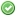rfc:array_key_first_last

# PHP RFC: array_key_first(), array_key_last() and array_value_first(), array_value_last()

## Introduction

As arrays are a powerful data structure it's handy in some cases to get the first or the last key/value of an array without a workaround. To accomplish this task this RFC adds four functions to the core:

• \$key = array_key_first(\$array);
• \$key = array_key_last(\$array);
• \$value = array_value_first(\$array);
• \$value = array_value_last(\$array);

## Proposal

The current functions of the PHP core only allow to retrieve the first/last key/value of an array by changing the internal state of the array when using the functions reset(), end() and key(). Other implementation approaches are either inperformant (eg. usage of array_keys(\$array) to gather the first key, which additionally may lead to errors if \$array is an empty array) or provide a construct which increases the cognitive complexity of the implementation (eg. usage of loops).

To avoid changes of the internal array representation in order to gather a single information from the array and to increase the comprehensibility of the userland code this RFC proposes new functions for this task. Two functions for the handling of array keys and two functions for the handling of array values:

To gather the key of the first element of an array the method array_key_first(\$array) will be added.

To gather the key of the last element of an array the method array_key_last(\$array) will be added.

The usage of the methods to handle the keys will look like:

```// usage of an associative array
\$array = ['a' => 1, 'b' => 2, 'c' => 3];

\$firstKey = array_key_first(\$array);
\$lastKey = array_key_last(\$array);

assert(\$firstKey === 'a');
assert(\$lastKey === 'c');

// usage of a numeric array
\$array = [1 => 'a', 2 => 'b', 3 => 'c'];

\$firstKey = array_key_first(\$array);
\$lastKey = array_key_last(\$array);

assert(\$firstKey === 1);
assert(\$lastKey === 3);

// usage of an empty array
\$array = [];

\$firstKey = array_key_first(\$array);
\$lastKey = array_key_last(\$array);

assert(\$firstKey === null);
assert(\$lastKey === null);```

To gather the value of the first element of an array the method array_value_first(\$array) will be added.

To gather the value of the last element of an array the method array_value_last(\$array) will be added.

The usage of the methods to handle the values will look like:

```// usage of an associative array
\$array = ['a' => 1, 'b' => 2, 'c' => 3];

\$firstValue = array_value_first(\$array);
\$lastValue = array_value_last(\$array);

assert(\$firstValue === 1);
assert(\$lastValue === 3);

// usage of a numeric array
\$array = [1 => 'a', 2 => 'b', 3 => 'c'];

\$firstValue = array_value_first(\$array);
\$lastValue = array_value_last(\$array);

assert(\$firstValue === 'a');
assert(\$lastValue === 'c');

// usage of an empty array
\$array = [];

\$firstValue = array_value_first(\$array);
\$lastValue = array_value_last(\$array);

assert(\$firstValue === null);
assert(\$lastValue === null);```

All four functions either return the requested key/value or null if an empty array is provided. If a non array parameter is given, a warning will be triggered and null will be returned.

None.

next PHP 7.x

## RFC Impact

As this RFC adds four new functions there is a possible impact on existing userland extensions where developers created helper functions with an identical name. As the functions proposed provide a meaningful name custom functions should implement an identical functionality and thus be replaceable.

There is no impact to SAPIs or the opcache.

## Vote

This is not a language change so a simple yes/no vote with 50%+1 majority is required.

Voting starts on 2018-07-09 13:30 UTC and closes on 2018-07-16 23:00 UTC.

Add array_key_first() and array_key_last()?
Real name Yes No
ajf (ajf)ashnazg (ashnazg)bwoebi (bwoebi)cmb (cmb)colinodell (colinodell)dams (dams)danack (danack)derick (derick)hywan (hywan)jhdxr (jhdxr)jwage (jwage)kalle (kalle)kelunik (kelunik)kguest (kguest)krakjoe (krakjoe)lbarnaud (lbarnaud)lcobucci (lcobucci)levim (levim)lex (lex)mcmic (mcmic)narf (narf)ocramius (ocramius)peehaa (peehaa)pmmaga (pmmaga)pollita (pollita)rtheunissen (rtheunissen)stas (stas)trowski (trowski)weierophinney (weierophinney)yohgaki (yohgaki)zeev (zeev)zimt (zimt)Final result: 18 14
This poll has been closed.

Add array_value_first() and array_value_last()?
Real name Yes No
ajf (ajf)ashnazg (ashnazg)bwoebi (bwoebi)cmb (cmb)colinodell (colinodell)dams (dams)danack (danack)derick (derick)hywan (hywan)jhdxr (jhdxr)jwage (jwage)kalle (kalle)kelunik (kelunik)kguest (kguest)krakjoe (krakjoe)lbarnaud (lbarnaud)lcobucci (lcobucci)levim (levim)lex (lex)mcmic (mcmic)nikic (nikic)ocramius (ocramius)peehaa (peehaa)pmmaga (pmmaga)pollita (pollita)rtheunissen (rtheunissen)stas (stas)svpernova09 (svpernova09)trowski (trowski)weierophinney (weierophinney)yohgaki (yohgaki)zeev (zeev)zimt (zimt)Final result: 15 18
This poll has been closed.

## Patches and Tests

The GitHub pull request which provides the functionality and the related tests is located at https://github.com/php/php-src/pull/3256

## Implementation

The implementation for array_key_first() and array_key_last() was merged for PHP 7.3 with the commit http://git.php.net/?p=php-src.git;a=commit;h=50516a6

The documentation for the added functions is loated at:

## History

### Version 1.1

• Extended the scope of this RFC to also cover functions for the handling of values to provide a complete function set for working with outer array elements.
rfc/array_key_first_last.txt · Last modified: 2018/07/18 13:06 by wol-soft

### Page Tools﻿